# Math in Focus Grade 5 Chapter 11 Practice 4 Answer Key Theoretical Probability and Experimental Probability

This handy Math in Focus Grade 5 Workbook Answer Key Chapter 11 Practice 4 Theoretical Probability and Experimental Probability provides detailed solutions for the textbook questions.

## Math in Focus Grade 5 Chapter 11 Practice 4 Answer Key Theoretical Probability and Experimental Probability

Use the table to answer the questions.
Express each probability as a decimal.

A spinner has four equal sections in four different colors, red, blue, green, and yellow. The spinner is spun 100 times. The table shows the number of times it lands on each color.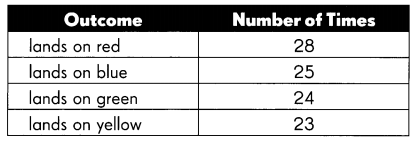Question 1.
What is the experimental probability of landing on red?
___________
$$\frac {28} {100}$$ = 0.28
Explanation:
Spinner is spun 100 times is denominator and spinner landing on red is 28 as numerator,
the fraction is the probability value.
As the probability is a number that reflects the chance or likelihood that a particular event will occur.
So red color lands 0.28 times.

Question 2.
What is the experimental probability of landing on blue?
___________
$$\frac {25} {100}$$ = 0.25
Explanation:
Spinner is spun 100 times is denominator and spinner landing on blue is 25 as numerator,
the fraction is the probability value.
As the probability is a number that reflects the chance or likelihood that a particular event will occur.
So blue color lands 0.25 times.

Question 3.
What is the experimental probability of landing on green?
___________
$$\frac {24} {100}$$ = 0.24
Explanation:
Spinner is spun 100 times is denominator and spinner landing on green is 24 as numerator,
the fraction is the probability value.
As the probability is a number that reflects the chance or likelihood that a particular event will occur.
So green color lands 0.24 times.

Question 4.
What is the experimental probability of landing on yellow?
___________
$$\frac {23} {100}$$ = 0.23
Explanation:
Spinner is spun 100 times is denominator and spinner landing on yellow is 23 as numerator,
the fraction is the probability value.
As the probability is a number that reflects the chance or likelihood that a particular event will occur.
So yellow color lands 0.23 times.

Question 5.
What is the theoretical probability of landing on each of the four colors?
___________
0.25 is the theoretical probability of landing on each of the four colors.
Explanation:
Spinner is spun 1 times as an experiment the spinner will stop at any one color out of four colors.
$$\frac {1} {4}$$ = 0.25
the fraction is the probability value.

Use the table to answer the questions.
Express each probability as a fraction is simplest form.

A number cube has 1 face numbered 1, 2 faces numbered 2, and 3 faces numbered 3. The cube is tossed 100 times. The table shows the number of times each number is shown.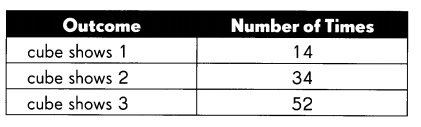Question 6.
What is the experimental probability of the cube showing 1?
___________
$$\frac {14} {100}$$ = 0.14
0.14 is the experimental probability of the cube showing 1.
Explanation:
The cube is tossed 100 times as an experiment the cube shows 1 is 14 times,
as six faces and 1 is on one  side only.
$$\frac {14} {100}$$ = 0.14
So, the fraction is the probability value.

Question 7.
What is the theoretical probability of the cube showing 1?
___________
0.16 is the theoretical probability of cube showing 1.
Explanation:
If the cube is tossed 1 time, the cube shows 1.
As there are six faces of cube, 1 is written on one side only.
$$\frac {1} {6}$$ = 0.16
So, the fraction is the probability value.

Question 8.
What is the experimental probability of the cube showing 2?
___________
0.34 is the experimental probability of cube showing 2.
Explanation:
The cube is tossed 100 times as an experiment the cube shows 2 is 34 times.
As six faces and 2 is on two sides only.
$$\frac {34} {100}$$ = 0.34
So, the fraction is the probability value.

Question 9.
What is the theoretical probability of the cube showing 2?
___________
0.33 the theoretical probability of the cube showing 2.
Explanation:
If the cube is tossed 1 time, the cube shows 2.
As there are six faces of cube, 2 is written on two sides.
$$\frac {2} {6}$$ = 0.33
So, the fraction is the probability value.

Question 10.
What is the experimental probability of the cube showing 3?
_______
0.52 is the experimental probability of cube showing 3.
Explanation:
The cube is tossed 100 times as an experiment the cube shows 3 is 52 times.
As six faces and 3 is on three sides only.
$$\frac {52} {100}$$ = 0.52
So, the fraction is the probability value.

Question 11.
What is the theoretical probability of the cube showing 3?
___________
0.5 the theoretical probability of the cube showing 3.
Explanation:
If the cube is tossed 1 time, the cube shows 3.
As there are six faces of cube, 3 is written on three sides.
$$\frac {3} {6}$$ = 0.5
So, the fraction is the probability value.

Use the table to answer the questions.
Express each probability as a decimal.

A bag contains 2 blue marbles, 3 red marbles, and 5 green marbles.
A marble is drawn from the bag, its color is noted and the marble is returned to the bag.
The table shows the results of drawing a marble 200 times.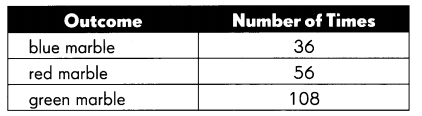Question 12.
What is the experimental probability of drawing a blue marble?
___________
0.18 is the experimental probability of drawing a blue marble
Explanation:
Given information is :
A bag contains 2 blue marbles, 3 red marbles, and 5 green marbles.
so, total marbles are 2 + 3 + 5 = 10
If a  marble is drawn from the bag, a blue marble as an experiment,
A blue marble is 36 out of 200 out comes.
$$\frac {36} {200}$$ = 0.18
So, the fraction is the probability value.

Question 13.
What is the theoretical probability of drawing a blue marble?
___________
0.2 is the theoretical probability of drawing a blue marble.
Explanation:
Given information is :
A bag contains 2 blue marbles, 3 red marbles, and 5 green marbles.
so, total marbles are 2 + 3 + 5 = 10
If a  marble is drawn from the bag, its color is either blue, red or green
Theoretical probability of drawing blue marble is $$\frac {2} {10}$$ = 0.2
So, the fraction is the probability value.

Question 14.
What is the experimental probability of drawing a red marble?
_______
0.28 is the experimental probability of drawing a red marble
Explanation:
Drawing a red marble as an experiment,
drawing a red marble is 56 out of 200 out comes.
$$\frac {56} {200}$$ = 0.28
So, the fraction is the probability value.

Question 15.
What is the theoretical probability of drawing a red marble?
___________
0.3 the theoretical probability of drawing a red marble.
Explanation:
Given information is :
A bag contains 2 blue marbles, 3 red marbles, and 5 green marbles.
so, total marbles are 2 + 3 + 5 = 10
If a  marble is drawn from the bag, its color is either blue, red or green.
Theoretical probability of drawing red marble is $$\frac {3} {10}$$ = 0.3
So, the fraction is the probability value.

Question 16.
What is the experimental probability of drawing a green marble?
___________
0.54 is the experimental probability of drawing a green marble.
Explanation:
Drawing a green marble as an experiment,
drawing a green marble is 108 out of 200 out comes.
$$\frac {108} {200}$$ = 0.54
So, the fraction is the probability value.

Question 17.
What is the theoretical probability of drawing a green marble?
___________
0.5 the theoretical probability of drawing a green marble.
Explanation:
Given information is
A bag contains 2 blue marbles, 3 red marbles, and 5 green marbles.
so, total marbles are 2 + 3 + 5 = 10
If a  marble is drawn from the bag, its color is either blue, red or green
Theoretical probability of drawing green marble is $$\frac {5} {10}$$ = 0.5
the fraction is the probability value

Complete.

A spinner is divided into 16 equal parts. Each part is colored green, yellow, or blue. The spinner is spun 25 times. The tally chart shows the number of times it lands on each color.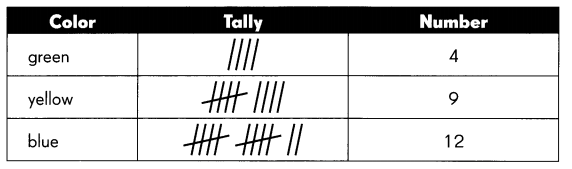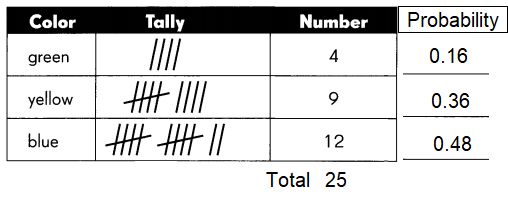Question 18.
Which is the likely set of colors on the spinner, Set A, Set B, or Set C?
Set ___________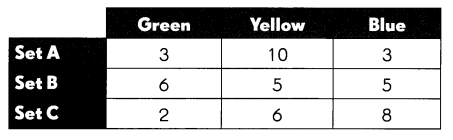Set C
Explanation:
green marble  $$\frac {2} {16}$$ = 0.125
yellow marble $$\frac {6} {16}$$ = 0.375
blue marble $$\frac {8} {16}$$ = 0.5

Question 19.
What is the experimental probability of landing on green?
_______
0.16 is is the experimental probability of landing on green.
Explanation:
Spinner is spun 25 times as an experiment as data shown in the above table,
the spinner will stop on green color 4 times out of 25 times experiment on three colors.
$$\frac {4} {25}$$ = 0.16
So, the fraction is the probability value.

Question 20.
What is the experimental probability of landing on yellow?
_______
0.36 is is the experimental probability of landing on yellow.
Explanation:
Spinner is spun 25 times as an experiment as data shown in the above table,
the spinner will stop on yellow color 9 times out of 25 times experiment on three colors.
$$\frac {9} {25}$$ = 0.36
So, the fraction is the probability value.

Question 21.
What is the experimental probability of landing on blue?
_______
$$\frac {12} {25}$$ = 0.48